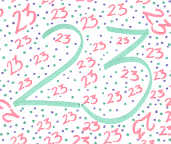# 23

This number is a prime.

Just showing those entries submitted by 'Teofilatto': (Click here to show all)Kristen Eller ©200223 is the only prime of the form p*q + p + q and p*q - p - q, where p and q are two successive primes (3*5 + 3 + 5 = 5*7 - 5 - 7 = 23). [Teofilatto]23 is the smallest and only prime of the form 2x^2 - y^2 and 3x^2 - y^2, where x and y are two consecutive numbers. [Teofilatto]23 is the smallest prime equal to the sum of three distinct primes in two ways: 5+7+11 = 3+7+13 = 23. [Teofilatto]23 = (2^2 + 3^3) - (2! + 3!). [Teofilatto]

(There is one curio for this number that has not yet been approved by an editor.)